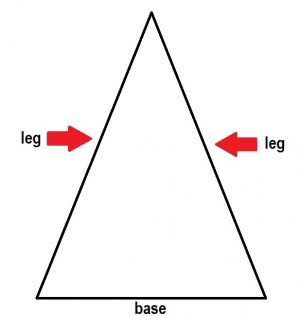# Ten unusual maths words

Mathematicians love to give everything a name. How many of the following unusual maths words have you heard of before?

### 1. Vinculum

Vinculum comes from Latin meaning to “bond or link”. A vinculum is a line which is used in mathematics above a group of numbers or symbols to show that they should be grouped together. It could be used in the following example situations:

• A vinculum is a line between the numerator and denominator in a fraction:$\frac{a}{b}$
• A vinculum is the line in a radical such as a square root:$\sqrt x$
• A vinculum can be used to show a group of repeating decimals:$0.\overline{142857}$

### 2. Oblate Spheroid

An Oblate Spheroid is a flattened sphere. In fact, this is the shape of the Earth!

Mathematically you can generate an Oblate Spheroid by rotating an ellipse around its minor (smallest) axis, making what is called a surface of revolution.### 3. Monomial

A monomial is an algebraic expression which only has one term. For example,$5x^2, 2x$, or$8$ are monomials.

A polynomial has many terms (two or more).

### 4. Lemma

In mathematics you get theorems (such as Pythagoras’ Theorem) but you also get Lemmas, which are often called ‘helping theorems’. They are not usually deemed to be important in their own right, they are smaller results which enable the proof of bigger results.

### 5. ‘Leg’ of an Isosceles Triangle

Not many people know that the technical name for each of the two same-length sides of an isosceles triangle is a ‘leg’.### 6. Googolplex

A Googol is the number$10^{100}$, and a Googolplex is the number$10^{Googol}$

The Googol has been made especially famous by the choice of name for the search engine Google.

The term Googol was however first invented in 1920 by a 9 year old boy called Milton Sirotta who was the nephew of mathematician Edward Kasner. Kasner went on to use the term Googol in his book Mathematics and the Imagination which was published in 1940.

### 7. Apothem

The apothem is the line segment which goes from the centre of a regular polygon to the midpoint of one of its sides.### 8. Ordinal numbers

Ordinal numbers represent position – for example 1st,2nd, 3rd. In contrast, cardinal numbers represent size or quantity: 1,2,3.

### 9. Abscissa

‘Abscissa’ is a fancy mathematical name for the x-coordinate, whereas the fancy mathematical name for the y -coordinate is the ‘ordinate’.

### 10. Camembert

This probably doesn’t count, given that it is a French word – however it is still interesting to know that the French word for Pie Chart is Camembert.Article by Hazel Lewis